# Two-phase flow in porous media: Analytical vs numerical solution (updated)

Update: 26 August 2021
We've been trying to use the analytical (Buckley-Leverett) solution of the two-phase flow in porous media to fit the Corey-type relative permeability model to the experimental oil recovery data. In this post, I'm going to compare the numerical solution of the same model with the analytical results. You can find the codes that I have written in this github repository. Here, I only call the codes and compare the results.

### Formulation¶

This document is mostly based on the SPE-7660 paper by Gary Pope. I first implement the simple water flooding analytical solution and then expand it to low salinity water flooding with and without ionic adsorption.

### Mathematical model¶

The two phase flow equation in a 1D porous medium reads $$\frac{\partial S_w}{\partial t}+\frac{u}{\varphi}\frac{df_w}{dS_w}\frac{\partial S_w}{\partial x} = 0$$ The dimensionless time and space are defined as $$t_D = \frac{ut}{\varphi L}$$ and $$x_D = \frac{x}{L}$$ The velocity of a constant saturation front is calculated by $$V_{S_w} = (\frac{dx}{dt})_{S_w}=\frac{u}{\varphi}\frac{df_w}{dS_w}$$ The shock front is specified by $$\frac{f_w(S_{w,shock})-f_w(S_{w,init})}{S_{w,shock}-S_{w,init}}=\left(\frac{df_w}{dS_w}\right)_{S_{w,shock}}$$ The injected water front velocity (i.e., a tracer in the injected water, or the low salinity of the injected brine) is calculated by $$V_{c} = (\frac{dx}{dt})_{c}=\frac{u}{\varphi}\frac{f_w}{S_w}$$ an the water saturation that corresponds to the position of the salinity front is given by $$\frac{f_w(S_{w,s})-f_w(0)}{S_{w}-0.0}=\left(\frac{df_w}{dS_w}\right)_{S_{w,shock}}$$ which is the tangent line fron the point (0,0) to the $f_w-S_w$ (fractional flow) curve. The breakthrough time (in number of pore volume) is calculated by $$t_{D, bt} = \left(\frac{df_w}{dS_w}\right)^{-1}_{S_{w,shock}}$$ The other useful relation is the average saturation after breakthrough, which reads $$S_{w,av} = S_{or}+\left[(1-f_w)\left(\frac{df_w}{dS_w}\right)\right]_{x=L}, \;t_D>t_{D,bt}$$ The recovery factor then can be calculated based on the fact that the recovery curve is linear until the breakthrough, and after breakthrough it gradually reaches a plateau. The oil recovery factor before the breakthrough is calculated by $$R = \frac{(1-f_w(S_{w,init}))t_D}{1-S_{w,init}}, \;t_D<t_{D,bt}$$ and after breakthrough by $$R = \frac{S_{w,init}-S_{w,av}}{1-S_{w,init}}, \; t_D>t_{D,bt}$$ Let's try the above formulation in Julia.

In :
include("../../../projects/peteng/analytical/FractionalFlow/FractionalFlow.jl")
using PyPlot
FF = FractionalFlow;

WARNING: replacing module FractionalFlow.


### Fractional flow package¶

This package can solve a number of multiphase flow problems in a 1D homogeneous porous medium in the absence of capillary pressure and gravity. The package has some convenience functions for data analysis and visualization. We can define and visualize relative permeability curves for oil and water as follows:

In :
rel_perms = FF.oil_water_rel_perms(krw0=0.4, kro0=0.9,
swc=0.15, sor=0.2, nw=2.0, no = 2.0)
FF.visualize(rel_perms)
FF.print_relperm(rel_perms, title="Corey rel-perm parameters")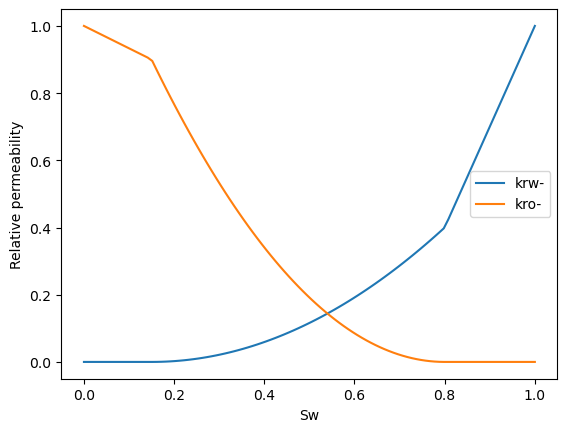Out:

#### Corey rel-perm parameters

krw0 kro0 nw no Swc Sor
0.4 0.9 2.0 2.0 0.15 0.2

Then we can construct the fractional flow curves:

In :
# define the fluids
fluids = FF.oil_water_fluids(mu_water=1e-3, mu_oil=2e-3)

# define the fractional flow functions
fw, dfw = FF.fractional_flow_function(rel_perms, fluids)
# visualize the fractional flow
FF.visualize(rel_perms, fluids, label="lowsal")
tight_layout()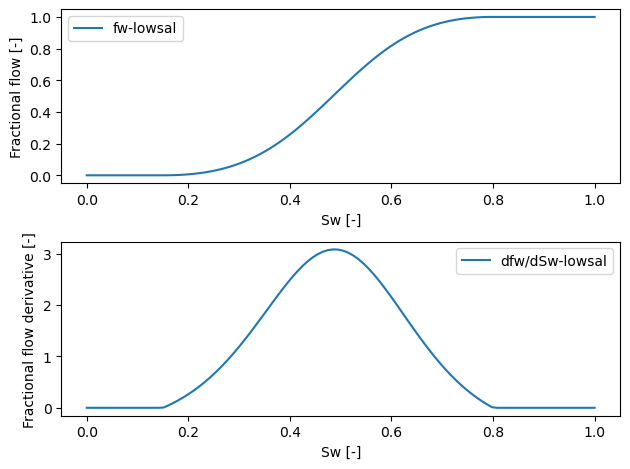The next stage is to define an injection problem, i.e. a core flooding test:

In :
core_flood = FF.core_flooding(u_inj=1.15e-5, pv_inject=5.0,
p_back=1e5, sw_init=0.2, sw_inj=1.0, rel_perms=rel_perms)
core_props = FF.core_properties()
wf_res = FF.water_flood(core_props, fluids, rel_perms, core_flood)
fw, dfw = FF.fractional_flow_function(rel_perms, fluids)
sw_tmp = range(0, 1, length=100)
FF.visualize(wf_res)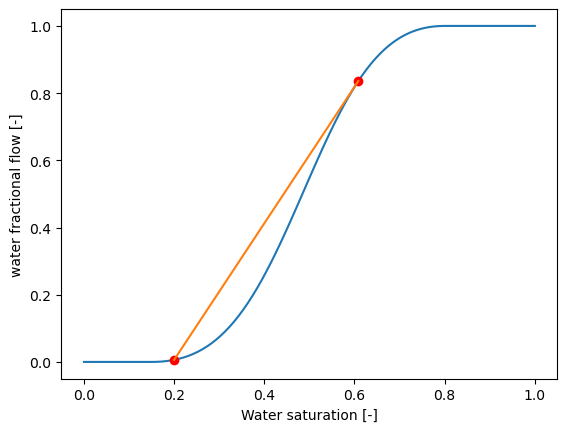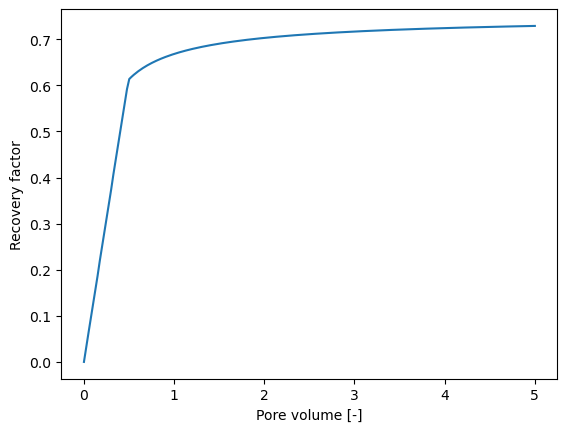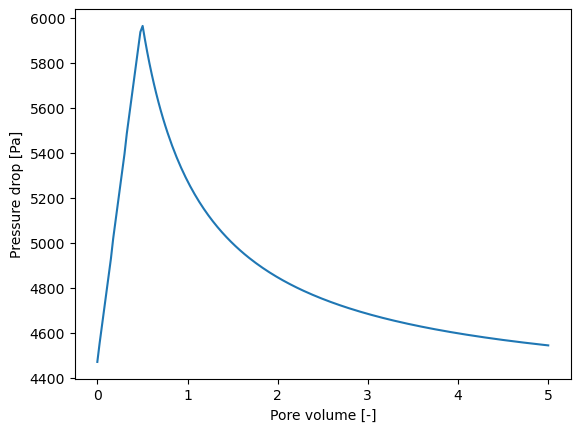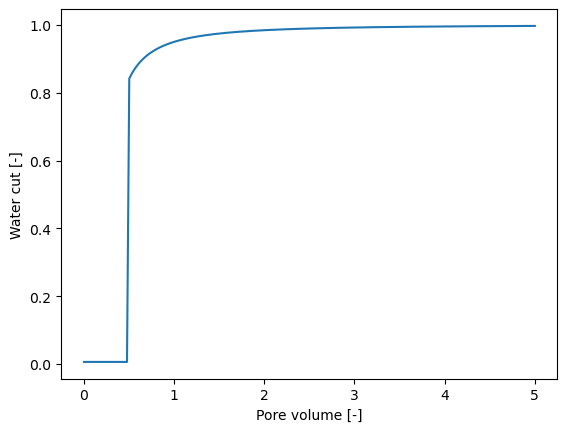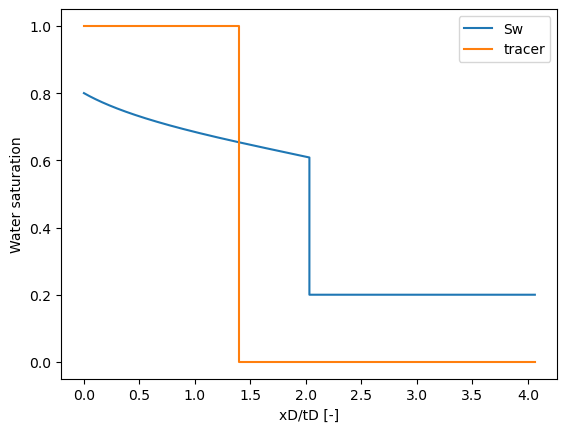Out:
PyObject <matplotlib.legend.Legend object at 0x7fe5f1169d90>

The same problem can be solved numerically as well. The code is reasonably fast and suitable for optimization:

In :
t_sec, pv_num, rec_fact, xt_num, sw_num, c_old, c_out_sal =
FF.water_flood_numeric(core_props, fluids, rel_perms,
core_flood, Nx = 20);

Progress: 100%|█████████████████████████████████████████| Time: 0:00:08


Let's have a look at the recovery factor versus time:

In :
figure(3)
plot(wf_res.recovery_pv[:,1], wf_res.recovery_pv[:,2], pv_num, rec_fact, "--")
legend(["Analytical", "Numerical"]);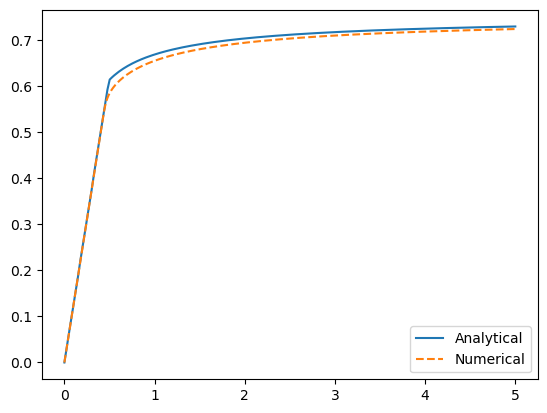One can see an underestimation of the recovery factor that is the result of the coarse mesh (Nx = 20). If I use a finer grid of, e.g. 500, the run time will be higher but my solution will be closer to the analytical one:

In :
t_sec_f, pv_num_f, rec_fact_f, xt_num_f, sw_num_f,
c_old_f, c_out_sal_f = FF.water_flood_numeric(
core_props, fluids, rel_perms, core_flood, Nx = 500
);

Progress: 100%|█████████████████████████████████████████| Time: 0:02:03

In :
figure(3)
plot(wf_res.recovery_pv[:,1], wf_res.recovery_pv[:,2], pv_num_f, rec_fact_f, "--")
legend(["Analytical", "Numerical (fine grid)"]);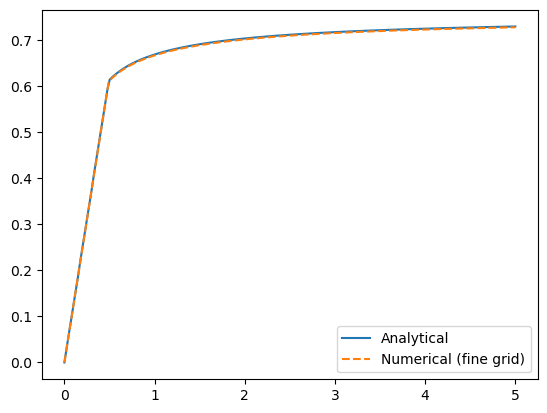It takes 2 minutes and seven seconds to run the code with a fine mesh versus 1 second with a coarse mesh. There are ways to make the code run faster on the fine mesh but at the end of the day we have to reach a compromise between the accuracy and speed based on our specific application for the numerical solution. I will write more about it later in the context of parameter estimation.